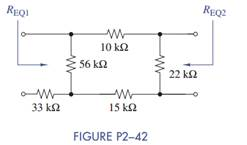Chat Now

# Equivalent resistance is defined at a particular pair of terminals. In Figure P2−42, the same...

### Equivalent resistance is defined at a particular pair of terminals. In Figure P2−42, the same...

Equivalent resistance is defined at a particular pair of terminals. In Figure P2−42, the same circuit is looked at from two different terminal pairs. Find the equivalent resistances REQ1 and REQ2 in Figure P2−42. Note that in calculating REQ2 the 33-kΩ resistor is connected to an open circuit and therefore does not affect the calculation.manish jayant 28-Jul-2020Get solution